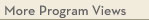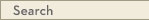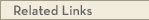# University Catalogs

Twin Cities campusTwin Cities Campus

# Statistics B.A.

Statistics, School of
College of Liberal Arts
• Students will no longer be accepted into this program after Spring 2017. Program requirements below are for current students only.
• See Statistical Practice BA
• Program Type: Baccalaureate
• Requirements for this program are current for Spring 2020
• Required credits to graduate with this degree: 120
• Required credits within the major: 51 to 55
• Degree: Bachelor of Arts
Statistics is concerned with theories and methods of data collection, tabulation, analysis, and interpretation, and their use in learning from data and making decisions. A bachelor's degree gives students an understanding of the theory of statistics and trains them in basic use of the most important types of statistical methods. The degree prepares students for graduate work or for jobs in diverse areas as marketing analysis, quality management, and support for scientific research.
Program Delivery
This program is available:
• via classroom (the majority of instruction is face-to-face)
General Requirements
All students in baccalaureate degree programs are required to complete general University and college requirements including writing and liberal education courses. For more information about University-wide requirements, see the liberal education requirements. Required courses for the major, minor or certificate in which a student receives a D grade (with or without plus or minus) do not count toward the major, minor or certificate (including transfer courses).
Program Requirements
Students are required to complete 4 semester(s) of any second language. with a grade of C-, or better, or S, or demonstrate proficiency in the language(s) as defined by the department or college.
Students must complete two years of mathematics, one course in computer programming, and 26 credits of STATS 3xxx or higher (two courses in statistical theory, two courses in statistical methods, at least three elective courses in statistics, and a senior project). Students may earn no more than one degree from the Statistics program: a B.A. or a B.S. or a minor. Beginning fall 2012, all incoming CLA freshmen must complete the appropriate First Year Experience course sequence. Specific information about this collegiate requirement can be found at: http://class.umn.edu/degree_requirements/index.html
Mathematics
Take a total of five courses for twenty credits.
MATH 1271 - Calculus I [MATH] (4.0 cr)
or MATH 1371 - CSE Calculus I [MATH] (4.0 cr)
MATH 1272 - Calculus II (4.0 cr)
or MATH 1372 - CSE Calculus II (4.0 cr)
MATH 2243 - Linear Algebra and Differential Equations (4.0 cr)
or MATH 2373 - CSE Linear Algebra and Differential Equations (4.0 cr)
MATH 2263 - Multivariable Calculus (4.0 cr)
or MATH 2374 - CSE Multivariable Calculus and Vector Analysis (4.0 cr)
MATH 4242 - Applied Linear Algebra (4.0 cr)
or Honors Sequence
MATH 1571H - Honors Calculus I [MATH] (4.0 cr)
MATH 1572H - Honors Calculus II (4.0 cr)
MATH 2573H - Honors Calculus III (4.0 cr)
MATH 2574H - Honors Calculus IV (4.0 cr)
MATH 4242 - Applied Linear Algebra (4.0 cr)
Major Courses
Take four courses for 15-16 credits.
STAT 3022 - Data Analysis (4.0 cr)
STAT 3011 - Introduction to Statistical Analysis [MATH] (4.0 cr)
or STAT 3021 - Introduction to Probability and Statistics (3.0 cr)
Choose a theory course group:
Option 1
STAT 4101 - Theory of Statistics I (4.0 cr)
STAT 4102 - Theory of Statistics II (4.0 cr)
or Option 2
STAT 5101 - Theory of Statistics I (4.0 cr)
STAT 5102 - Introduction to Statistical Learning (4.0 cr)
or Option 3
MATH 5651 - Basic Theory of Probability and Statistics (4.0 cr)
STAT 5102 - Introduction to Statistical Learning (4.0 cr)
Programming for Statisticians
Take one course for 4 credits.
CSCI 1103 - Introduction to Computer Programming in Java (4.0 cr)
or CSCI 1113 - Introduction to C/C++ Programming for Scientists and Engineers (4.0 cr)
or CSCI 1913 - Introduction to Algorithms, Data Structures, and Program Development (4.0 cr)
or CSCI 1933 - Introduction to Algorithms and Data Structures (4.0 cr)
Electives
Take 3 or more course(s) totaling 9 - 12 credit(s) from the following:
· STAT 5031 - Statistical Methods for Quality Improvement (4.0 cr)
· STAT 5041 {Inactive} (3.0 cr)
· STAT 5201 - Sampling Methodology in Finite Populations (3.0 cr)
· STAT 5302 - Applied Regression Analysis (4.0 cr)
· STAT 5303 - Designing Experiments (4.0 cr)
· STAT 5401 - Applied Multivariate Methods (3.0 cr)
· STAT 5421 - Analysis of Categorical Data (3.0 cr)
· STAT 5511 - Time Series Analysis (3.0 cr)
· STAT 5601 - Nonparametric Methods (3.0 cr)
· STAT 5931 - Topics in Statistics (3.0 cr)
· STAT 5932 {Inactive} (3.0 cr)
Senior Project
STAT 4893W - Consultation and Communication for Statisticians [WI] (3.0 cr)
Upper-division Writing Intensive within the major
Students are required to take one upper-division Writing Intensive course within the major. If that requirement has not been satisfied within the core major requirements, students must choose one course from the following list. Some of these courses may also fulfill other major requirements.
Take 0 - 1 course(s) from the following:
· STAT 4893W - Consultation and Communication for Statisticians [WI] (3.0 cr)View college catalog(s): · College of Liberal Arts View sample plan(s): · Statistics View checkpoint chart: · Statistics B.A. View PDF Version:Search Programs Search University CatalogsOne Stop for tuition, course registration, financial aid, academic calendars, and moreMATH 1271 - Calculus I (MATH)
 Credits: 4.0 [max 4.0] Course Equivalencies: 00067 - Math 1271/Math 1281/Math 1371/ Typically offered: Every Fall, Spring & Summer
Differential calculus of functions of a single variable, including polynomial, rational, exponential, and trig functions. Applications, including optimization and related rates problems. Single variable integral calculus, using anti-derivatives and simple substitution. Applications may include area, volume, work problems. prereq: 4 yrs high school math including trig or satisfactory score on placement test or grade of at least C- in [1151 or 1155]
MATH 1371 - CSE Calculus I (MATH)
 Credits: 4.0 [max 4.0] Course Equivalencies: Math 1142/1271/1281/1371/1571H Typically offered: Every Fall & Spring
Differentiation of single-variable functions, basics of integration of single-variable functions. Applications: max-min, related rates, area, curve-sketching. Use of calculator, cooperative learning. prereq: CSE or pre-bioprod concurrent registration is required (or allowed) in biosys engn (PRE), background in [precalculus, geometry, visualization of functions/graphs], instr consent; familiarity with graphing calculators recommended
MATH 1272 - Calculus II
 Credits: 4.0 [max 4.0] Course Equivalencies: Math 1272/1282/1252/1372/1572 Typically offered: Every Fall, Spring & Summer
Techniques of integration. Calculus involving transcendental functions, polar coordinates. Taylor polynomials, vectors/curves in space, cylindrical/spherical coordinates. prereq: [1271 or equiv] with grade of at least C-
MATH 1372 - CSE Calculus II
 Credits: 4.0 [max 4.0] Course Equivalencies: Math 1272/1282/1252/1372/1572 Typically offered: Every Spring
Techniques of integration. Calculus involving transcendental functions, polar coordinates, Taylor polynomials, vectors/curves in space, cylindrical/spherical coordinates. Use of calculators, cooperative learning. prereq: Grade of at least C- in [1371 or equiv], CSE or pre-Bioprod/Biosys Engr
MATH 2243 - Linear Algebra and Differential Equations
 Credits: 4.0 [max 4.0] Course Equivalencies: Math 2243/2373/2573 Typically offered: Every Fall, Spring & Summer
Linear algebra: basis, dimension, matrices, eigenvalues/eigenvectors. Differential equations: first-order linear, separable; second-order linear with constant coefficients; linear systems with constant coefficients. prereq: [1272 or 1282 or 1372 or 1572] w/grade of at least C-
MATH 2373 - CSE Linear Algebra and Differential Equations
 Credits: 4.0 [max 4.0] Course Equivalencies: Math 2243/2373/2573 Typically offered: Every Fall & Spring
Linear algebra: basis, dimension, eigenvalues/eigenvectors. Differential equations: linear equations/systems, phase space, forcing/resonance, qualitative/numerical analysis of nonlinear systems, Laplace transforms. Use of computer technology. prereq: [1272 or 1282 or 1372 or 1572] w/grade of at least C-, CSE or pre-Bio Prod/Biosys Engr
MATH 2263 - Multivariable Calculus
 Credits: 4.0 [max 4.0] Course Equivalencies: Math 2263/2373/2573H/3251 Typically offered: Every Fall, Spring & Summer
Derivative as linear map. Differential/integral calculus of functions of several variables, including change of coordinates using Jacobians. Line/surface integrals. Gauss, Green, Stokes Theorems. prereq: [1272 or 1372 or 1572] w/grade of at least C-
MATH 2374 - CSE Multivariable Calculus and Vector Analysis
 Credits: 4.0 [max 4.0] Course Equivalencies: Math 2263/2373/2573H/3251 Typically offered: Every Fall & Spring
Derivative as linear map. Differential/integral calculus of functions of several variables, including change of coordinates using Jacobians. Line/surface integrals. Gauss, Green, Stokes theorems. Use of computer technology. prereq: [1272 or 1282 or 1372 or 1572] w/grade of at least C-, CSE or pre-Bioprod/Biosys Engr
MATH 4242 - Applied Linear Algebra
 Credits: 4.0 [max 4.0] Course Equivalencies: 01212 - Math 4242/Math 4457 Typically offered: Every Fall, Spring & Summer
Systems of linear equations, vector spaces, subspaces, bases, linear transformations, matrices, determinants, eigenvalues, canonical forms, quadratic forms, applications. prereq: 2243 or 2373 or 2573
MATH 1571H - Honors Calculus I (MATH)
 Credits: 4.0 [max 4.0] Course Equivalencies: 00067 - Math 1142/1271/1281/1371/1571H Grading Basis: A-F only Typically offered: Every Fall
Differential/integral calculus of functions of a single variable. Emphasizes hard problem-solving rather than theory. prereq: Honors student and permission of University Honors Program
MATH 1572H - Honors Calculus II
 Credits: 4.0 [max 4.0] Course Equivalencies: 00068 - Math 1272/1282/1252/1372/1572 Grading Basis: A-F only Typically offered: Every Spring
Continuation of 1571. Infinite series, differential calculus of several variables, introduction to linear algebra. prereq: 1571H, honors student, permission of University Honors Program
MATH 2573H - Honors Calculus III
 Credits: 4.0 [max 4.0] Course Equivalencies: 00069 - Math 2263/2374/3251 Grading Basis: A-F only Typically offered: Every Fall
Integral calculus of several variables. Vector analysis, including theorems of Gauss, Green, Stokes. prereq: Math 1572H or Math 2574H, honors student and permission of University Honors Program
MATH 2574H - Honors Calculus IV
 Credits: 4.0 [max 4.0] Course Equivalencies: 00561 - Math 2243/Math 2373/Math 2573H Grading Basis: A-F only Typically offered: Every Spring
Advanced linear algebra, differential equations. Additional topics as time permits. prereq: Math 1572H or Math 2573H, honors student and permission of University Honors Program
MATH 4242 - Applied Linear Algebra
 Credits: 4.0 [max 4.0] Course Equivalencies: 01212 - Math 4242/Math 4457 Typically offered: Every Fall, Spring & Summer
Systems of linear equations, vector spaces, subspaces, bases, linear transformations, matrices, determinants, eigenvalues, canonical forms, quadratic forms, applications. prereq: 2243 or 2373 or 2573
STAT 3022 - Data Analysis
 Credits: 4.0 [max 4.0] Typically offered: Every Fall & Spring
Practical survey of applied statistical inference/computing covering widely used statistical tools. Multiple regression, variance analysis, experiment design, nonparametric methods, model checking/selection, variable transformation, categorical data analysis, logistic regression. prereq: 3011 or 3021 or SOC 3811
STAT 3011 - Introduction to Statistical Analysis (MATH)
 Credits: 4.0 [max 4.0] Course Equivalencies: (Select a set) Typically offered: Every Fall, Spring & Summer
Standard statistical reasoning. Simple statistical methods. Social/physical sciences. Mathematical reasoning behind facts in daily news. Basic computing environment.
STAT 3021 - Introduction to Probability and Statistics
 Credits: 3.0 [max 3.0] Typically offered: Every Fall, Spring & Summer
This is an introductory course in statistics whose primary objectives are to teach students the theory of elementary probability theory and an introduction to the elements of statistical inference, including testing, estimation, and confidence statements. prereq: Math 1272
STAT 4101 - Theory of Statistics I
 Credits: 4.0 [max 4.0] Typically offered: Every Fall
Random variables/distributions. Generating functions. Standard distribution families. Data summaries. Sampling distributions. Likelihood/sufficiency. prereq: Math 1272 or Math 1372 or Math 1572H
STAT 4102 - Theory of Statistics II
 Credits: 4.0 [max 4.0] Course Equivalencies: 00260 Typically offered: Every Spring
Estimation. Significance tests. Distribution free methods. Power. Application to regression and to analysis of variance/count data. prereq: STAT 4101
STAT 5101 - Theory of Statistics I
 Credits: 4.0 [max 4.0] Typically offered: Every Fall
Logical development of probability, basic issues in statistics. Probability spaces. Random variables, their distributions and expected values. Law of large numbers, central limit theorem, generating functions, multivariate normal distribution. prereq: [Math 2263 or Math 2374 or Math 2573H], [CSCI 2033 or Math 2373 or Math 2243]
STAT 5102 - Introduction to Statistical Learning
 Credits: 4.0 [max 4.0] Course Equivalencies: 00260 Typically offered: Every Spring
Sampling, sufficiency, estimation, test of hypotheses, size/power. Categorical data. Contingency tables. Linear models. Decision theory. prereq: [5101 or Math 5651 or instr consent]
MATH 5651 - Basic Theory of Probability and Statistics
 Credits: 4.0 [max 4.0] Course Equivalencies: 00259 - MATH 4653/Math 5651/Stat 5101 Typically offered: Every Fall & Spring
Logical development of probability, basic issues in statistics. Probability spaces, random variables, their distributions/expected values. Law of large numbers, central limit theorem, generating functions, sampling, sufficiency, estimation. prereq: [2263 or 2374 or 2573], [2243 or 2373]; [2283 or 2574 or 3283] recommended.
STAT 5102 - Introduction to Statistical Learning
 Credits: 4.0 [max 4.0] Course Equivalencies: 00260 Typically offered: Every Spring
Sampling, sufficiency, estimation, test of hypotheses, size/power. Categorical data. Contingency tables. Linear models. Decision theory. prereq: [5101 or Math 5651 or instr consent]
CSCI 1103 - Introduction to Computer Programming in Java
 Credits: 4.0 [max 4.0] Typically offered: Every Fall
Fundamental programming concepts/software development using Java language. Problem solving skills. Algorithm development techniques. Use of abstractions/modularity. Data structures/abstract data types. Substantial programming projects. Weekly lab.
CSCI 1113 - Introduction to C/C++ Programming for Scientists and Engineers
 Credits: 4.0 [max 4.0] Typically offered: Every Fall, Spring & Summer
Programming for scientists/engineers. C/C++ programming constructs, object-oriented programming, software development, fundamental numerical techniques. Exercises/examples from various scientific fields. prereq: Math 1271 or Math 1371 or Math 1571H or instr consent
CSCI 1913 - Introduction to Algorithms, Data Structures, and Program Development
 Credits: 4.0 [max 4.0] Typically offered: Every Fall, Spring & Summer
Advanced object oriented programming to implement abstract data types(stacks, queues, linked lists, hash tables, binary trees) using Java language. Searching/sorting algorithms. Basic algorithmic analysis. Scripting languages using Python language. Substantial programming projects. Weekly lab. prereq: (EE major and EE 1301) or (CmpE major and EE 1301) or 1103 or 1113 or instr consent
CSCI 1933 - Introduction to Algorithms and Data Structures
 Credits: 4.0 [max 4.0] Course Equivalencies: 00008 Typically offered: Every Fall, Spring & Summer
Advanced object oriented programming to implement abstract data types (stacks, queues, linked lists, hash tables, binary trees) using Java language. Inheritance. Searching/sorting algorithms. Basic algorithmic analysis. Use of software development tools. Weekly lab. prereq: 1133 or instr consent
STAT 5031 - Statistical Methods for Quality Improvement
 Credits: 4.0 [max 4.0] Typically offered: Periodic Spring
Random variability/sampling. Controlling statistical process. Shewhart/accumulative charting. Analyzing plant data, trend surface, and variance/design of experiments. prereq: [3021 or 3022 or 4102 or 5021 or 5102 or 8102], Math 1272
STAT 5201 - Sampling Methodology in Finite Populations
 Credits: 3.0 [max 3.0] Typically offered: Every Spring
Simple random, systematic, stratified, unequal probability sampling. Ratio, model based estimation. Single stage, multistage, adaptive cluster sampling. Spatial sampling. prereq: 3022 or 4102 or 5021 or 5102 or instr consent
STAT 5302 - Applied Regression Analysis
 Credits: 4.0 [max 4.0] Typically offered: Every Fall, Spring & Summer
Simple, multiple, and polynomial regression. Estimation, testing, prediction. Use of graphics in regression. Stepwise and other numerical methods. Weighted least squares, nonlinear models, response surfaces. Experimental research/applications. prereq: 3032 or 3022 or 4102 or 5021 or 5102 or instr consent
STAT 5303 - Designing Experiments
 Credits: 4.0 [max 4.0] Typically offered: Every Fall, Spring & Summer
Analysis of variance. Multiple comparisons. Variance-stabilizing transformations. Contrasts. Construction/analysis of complete/incomplete block designs. Fractional factorial designs. Confounding split plots. Response surface design. prereq: 3022 or 4102 or 5021 or 5102 or instr consent
STAT 5401 - Applied Multivariate Methods
 Credits: 3.0 [max 3.0] Typically offered: Periodic Fall
Bivariate and multivariate distributions. Multivariate normal distributions. Analysis of multivariate linear models. Repeated measures, growth curve and profile analysis. Canonical correlation analysis. Principal components and factor analysis. Discrimination, classification, and clustering.
STAT 5421 - Analysis of Categorical Data
 Credits: 3.0 [max 3.0] Typically offered: Every Fall & Spring
Varieties of categorical data, cross-classifications, contingency tables. Tests for independence. Combining 2x2 tables. Multidimensional tables/loglinear models. Maximum-likelihood estimation. Tests for goodness of fit. Logistic regression. Generalized linear/multinomial-response models.
STAT 5511 - Time Series Analysis
 Credits: 3.0 [max 3.0] Typically offered: Every Fall
Characteristics of time series. Stationarity. Second-order descriptions, time-domain representation, ARIMA/GARCH models. Frequency domain representation. Univariate/multivariate time series analysis. Periodograms, non parametric spectral estimation. State-space models. prereq: Theoretical understanding, STAT 4102 or STAT 5102
STAT 5601 - Nonparametric Methods
 Credits: 3.0 [max 3.0] Typically offered: Every Fall & Spring
Order statistics. Classical rank-based procedures (e.g., Wilcoxon, Kruskal-Wallis). Goodness of fit. Topics may include smoothing, bootstrap, and generalized linear models. prereq: Stat classes 3032 or 3022 or 4102 or 5021 or 5102 or instr consent
STAT 5931 - Topics in Statistics
 Credits: 3.0 [max 3.0] Typically offered: Periodic Fall
Topics vary according to student needs and available staff.
STAT 4893W - Consultation and Communication for Statisticians (WI)
 Credits: 3.0 [max 3.0] Prerequisites: Senior Statistics Major Grading Basis: A-F only Typically offered: Every Fall & Spring
This course focuses on how to interact and collaborate as a statistician on a multidisciplinary team. Students will learn about all aspects of statistical consulting by performing an actual consultation. This includes: understanding the needs of the researcher, designing a study to investigate the client's needs, and communicating study results through graphs, writing, and oral presentations in a manner that a non-statistician can understand. Students will also discuss how to design research ethically (respecting the rights of the subjects in the research), how to analyze data without manipulating results, and how to properly cite and credit other people's work. Students will also be exposed to professional statisticians as a means of better understanding careers in statistics. prereq: Senior Statistics Major
STAT 4893W - Consultation and Communication for Statisticians (WI)
 Credits: 3.0 [max 3.0] Prerequisites: Senior Statistics Major Grading Basis: A-F only Typically offered: Every Fall & Spring
This course focuses on how to interact and collaborate as a statistician on a multidisciplinary team. Students will learn about all aspects of statistical consulting by performing an actual consultation. This includes: understanding the needs of the researcher, designing a study to investigate the client's needs, and communicating study results through graphs, writing, and oral presentations in a manner that a non-statistician can understand. Students will also discuss how to design research ethically (respecting the rights of the subjects in the research), how to analyze data without manipulating results, and how to properly cite and credit other people's work. Students will also be exposed to professional statisticians as a means of better understanding careers in statistics. prereq: Senior Statistics Major